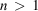International
Tables for
Crystallography
Volume B
Reciprocal space
Edited by U. Shmueli

International Tables for Crystallography (2010). Vol. B, ch. 1.3, pp. 32-33   | 1 | 2 |

## Section 1.3.2.3.9.4. Division of distributions by functions

G. Bricognea

aGlobal Phasing Ltd, Sheraton House, Suites 14–16, Castle Park, Cambridge CB3 0AX, England, and LURE, Bâtiment 209D, Université Paris-Sud, 91405 Orsay, France

#### 1.3.2.3.9.4. Division of distributions by functions

| top | pdf |

Given a distribution S onand an infinitely differentiable multiplier function α, the division problem consists in finding a distribution T such that.

If α never vanishes,is the unique answer. If, and if α has only isolated zeros of finite order, it can be reduced to a collection of cases where the multiplier is, for which the general solution can be shown to be of the formwhere U is a particular solution of the division problemand theare arbitrary constants.

In dimension, the problem is much more difficult, but is of fundamental importance in the theory of linear partial differential equations, since the Fourier transformation turns the problem of solving these into a division problem for distributions [see Hörmander (1963)].

### References

Hörmander, L. (1963). Linear Partial Differential Operators. Berlin: Springer-Verlag.